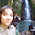## Monday, September 12, 2016

### Note: Parallelization not working with Maxent models

For the time being and for the foreseeable future, Maxent models aren't working with multiple cores.  This is due to an issue with the mclapply and rJava functions in R; rJava just straight-up does not work with mclapply, and as far as I can tell there's no way to make it do so.  As it stands, ENMTools just sets the number of cores to 1 for any of the tests when "type" is set to "mx".  If anyone knows, or discovers, a workaround for this please do let me know!

1.This comment has been removed by the author.

2.I did the identity.test with multiple cores using maxent type. We create a list with the occurrences for each core, so the replicates will be doing divided. Apparently it works, because the empirical value was the same for all replicates, I just need to calculate the p value again join all these matrices of replicates.
list_sp1<-vector("list",11)
for(i in 1:11){
list_sp1[[i]]<-data.sp1
}
library(parallel)
cl1<- makeCluster(11)
clusterExport(cl1,c("list_sp1","data.sp2","variables"))
clusterEvalQ(cl1,library(ENMTools))
###ID test
res<-parLapply(cl1,list_sp1,function(i) identity.test(species.1 = i,species.2 = data.sp2,
env = variables, type = "mx", nreps = 9))
stopCluster(cl1)
###For calculate p value D:
obsD<-res[]\$reps.overlap[1,1]
reps_list_D<-vector("list",length(res))
for(i in 1:length(res)){
reps_list_D[[i]]<-res[[i]]\$reps.overlap[-1,c(1)]
}
reps_D<-sort(unlist(reps_list_D))
replicate_D<-matrix(reps_D,nrow=length(reps_D),ncol=1,dimnames=list(c(1:length(reps_D)),c("D")),byrow = FALSE)
-1*(sum(replicate_D>=obsD)/(length(replicate_D)+1)-1)
###the same for for I index